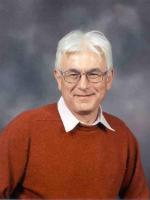# Faculty of MathematicsResearch Interests: Dynamical systems, statistical mechanics (classical and quantum), queueing networks, random processes and fields

## Publications

An Ising interface between two walls: Competition between two tendencies
JL Lebowitz, AE Mazel, YM Suhov
– Reviews in Mathematical Physics
(2011)
8,
669
Gibbs measures for SOS models on a Cayley tree
UA Rozikov, YM Suhov
– Infinite Dimensional Analysis Quantum Probability and Related Topics
(2011)
9,
471
Dynamical localization for a multi-particle model with an alloy-type external random potential
V Chulaevsky, AB de Monvel, Y Suhov
– Nonlinearity
(2011)
24,
1451
Anderson localization for a multi-particle model with alloy-type external potential
ABD Monvel, V Chulaevsky, P Stollmann, Y Suhov
(2010)
Wegner-type Bounds for a Multi-particle Continuous Anderson Model with an Alloy-type External Potential
AB de Monvel, V Chulaevsky, P Stollmann, Y Suhov
– Journal of Statistical Physics
(2009)
138,
553
Anderson localization in a two-particle continuous model with an alloy-type external potential
ABD Monvel, V Chulaevsky, P Stollmann, Y Suhov
(2009)
Multi-particle Anderson Localisation: Induction on the Number of Particles
V Chulaevsky, Y Suhov
– Mathematical Physics, Analysis and Geometry
(2009)
12,
117
Eigenfunctions in a Two-Particle Anderson Tight Binding Model
V Chulaevsky, Y Suhov
– Communications in Mathematical Physics
(2009)
289,
701
Wegner-type bounds for a two-particle Anderson model in a continuous space
ABD Monvel, V Chulaevsky, Y Suhov
(2008)
Large-Time Behavior of a Branching Diffusion on a Hyperbolic Space
M Kelbert, Y Suhov
– Theory of Probability and Its Applications
(2008)
52,
594
• 1 of 6
• >

## Research Groups

DPMMS retired
Probability
Applied Probability
Mathematical Finance and Operational Research
Statistical Laboratory

D0.24

01223 337964